﻿ Solution of P versus NP problemAlgorithms Research

p-ISSN: 2324-9978    e-ISSN: 2324-996X

2015;  4(1): 1-7

doi:10.5923/j.algorithms.20150401.01

### Solution of P versus NP problem

Mustapha Hamidi

Meknes, Morocco

Correspondence to: Mustapha Hamidi , Meknes, Morocco.
 Email: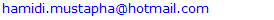Abstract

This paper, taking Travelling Salesman Problem as our object, wishes to develop a constructive algorithm to prove P=NP. Our algorithm is described as this process: initially, constructing a convex polygon that includes all points and creating a special centroid of this convex polygon, next, examine the position of each point compared to the centroid's position; then, repeating the previous step until all vertexes are included into the optimal tour. This paper has shown that our constructive algorithm can solve the Travelling Salesman Problem in polynomial time.

Keywords: NP-complete problems, Travelling salesman problem, Algorithm, Distance, Point, Convex polygon, Triangle, Special Centroid

Cite this paper: Mustapha Hamidi , Solution of P versus NP problem, Algorithms Research , Vol. 4 No. 1, 2015, pp. 1-7. doi: 10.5923/j.algorithms.20150401.01.

### 1. Introduction

This paper, taking Travelling Salesman Problem as our object, wishes to develop a constructive algorithm to prove P=NP, which is one of the seven Millennium Prize Problems selected by the Clay Mathematics Institute, and is also a major unsolved problem in computer science. NP represents the class of questions for which there is no known way to find an answer quickly, but an answer can be verified in polynomial time. For the hardest NP problems (i.e., NP-complete problems), given an efficient algorithm for any one of them, we can find an efficient algorithm for all of them [1-4].
The Traveling Salesman Problem (TSP) is the problem of finding a least-cost sequence in which to visit a set of cities, starting and ending at the same city, and in such a way that each city is visited exactly once. This problem has received a tremendous amount of attention over the years due in part to its wide applicability in practice (see , for examples). Also, since its seminal formulation as a mathematical programming problem in the 1950's , the problem has been at the core of most of the developments in the area of Combinatorial Optimization . A key issue has been the question of whether there exists a polynomial-time algorithm for solving the problem .
In this paper, we present a polynomial-sized linear programming formulation of the Traveling Salesman Problem (TSP).Figure 1. Euler diagram for P, NP, NP-complete, and NP-hard set of problems

### 2. Solution’s Idea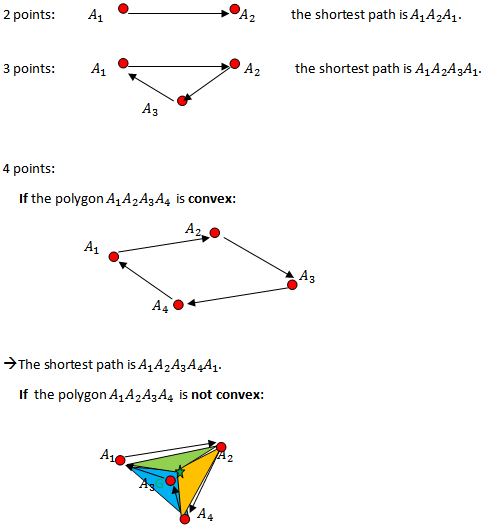Let G be the special centroid of triangle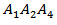, which verifies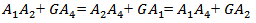We have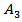within the triangle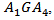and thus the shortest distance is obtained by connectingto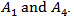àTherefore the shortest path is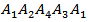.
It describes the main concept of the solution.

### 3. Algorithm

Let n be a number of points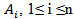; and let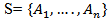be a set of these points.
We want to calculate the minimum distance between these points by returning to the starting point.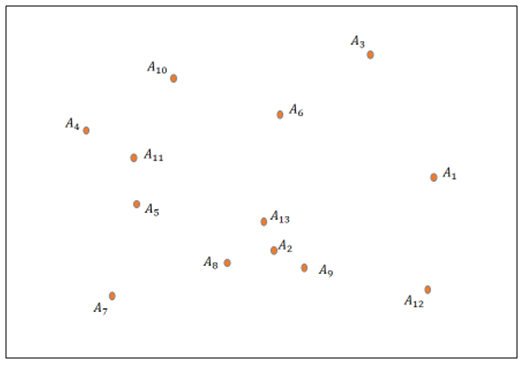Figure 2. Distribution of points for which we want to calculate a minimum distance by returning to the starting point
First, we create a convex polygon that includes all points by the “look left”, method .
Creation of convex polygon: look left:
We will examine our points in order, as if we start from one point, and continue by driving around our polygon. The idea is to choose the correct direction so that the interior of the polygon is to the left.
Here is the concept:
A point is inside the polygon if and only if it is "on your left" all along your route. Thus for each orientation as we proceed around the polygon, we examine whether the point being tested is to the left or not. If it is to the right, even once, then the point is not inside.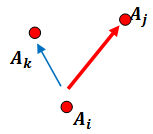Determinant:
Mathematically, a simple calculation suffices as the determinant.
We have points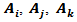.
Let D be the vector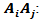Determining the polygon containing the points of the set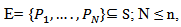where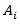gives :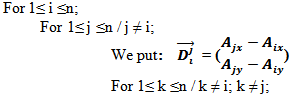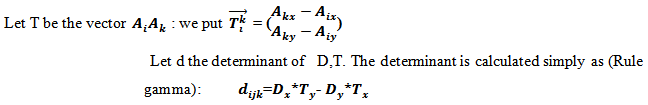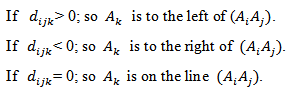Thus the convex polygon is as shown in Figure 3.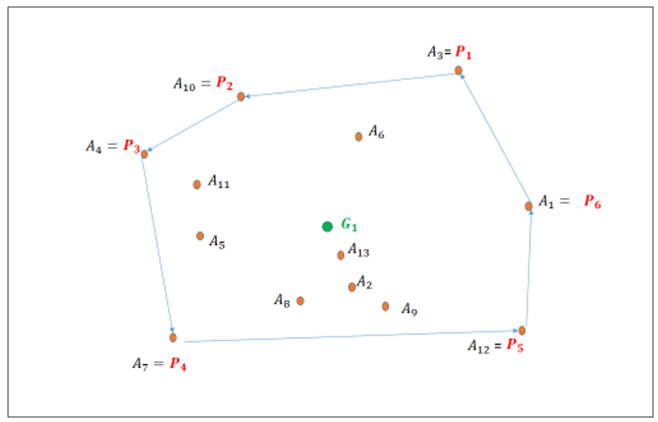Figure 3. Creation of convex polygon that includes all points
Thus, let N be the number of points in the convex polygon, and let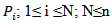are the points belonging to the convex polygon.
Let G be the special centroid of the polygon, which verifies: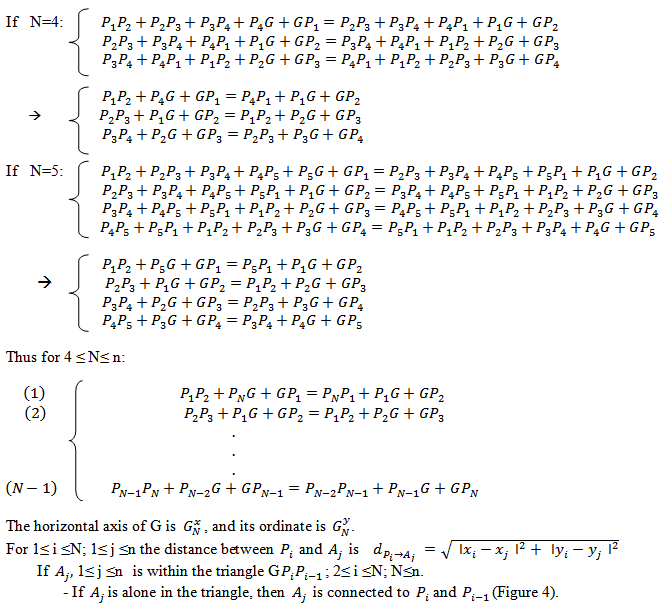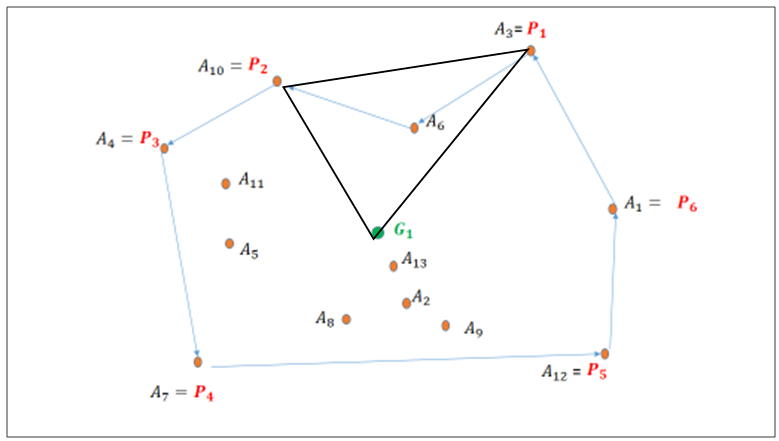Figure 4. One point in the triangle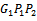- If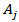is alone in the triangle, thenis connected to(Figure 4).
- If there are two pointsand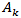, 1≤ j ≤ n and 1≤ k ≤ n within the triangle (Figure 5), then:
- If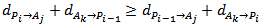thenis connected to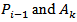.
- Otherwisewill be connected to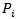and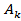.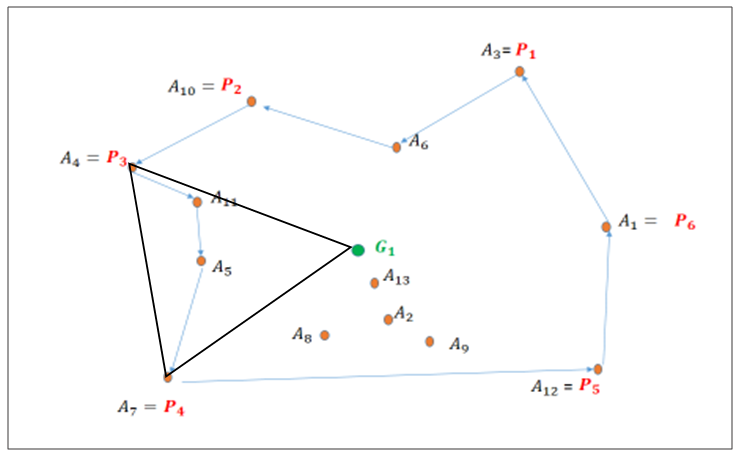Figure 5. Two points in the triangle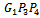- If there are r pointswithin the triangle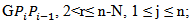we compose the convex polygon between
the points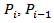that includes points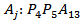(Figure 6).
Therefore, there are M points forming a set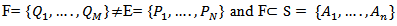.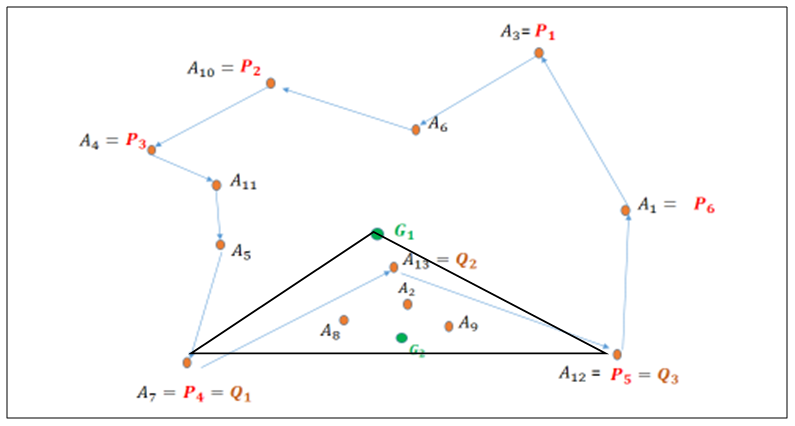Figure 6. Many points in the triangle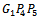, thus we create a new convex polygon within the triangle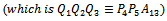and its centroid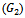We are looking for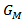, a centroid of M points, and apply the same method as above (from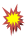) until all point
of triangle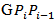are included into the optimal tour, and then proceed to point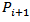.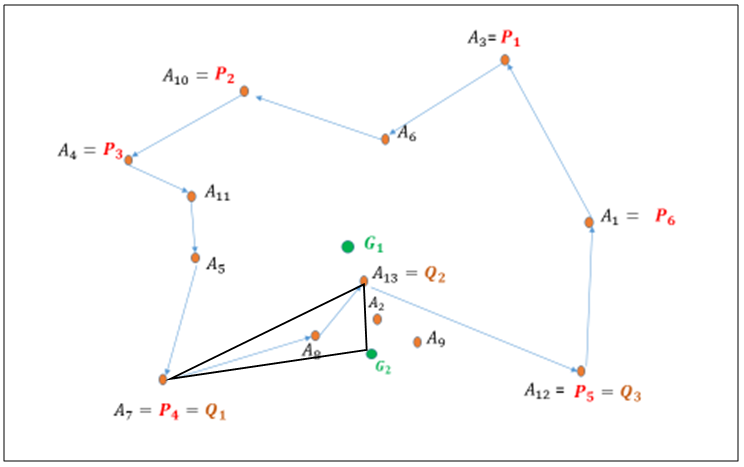Figure 7. One point in the triangle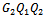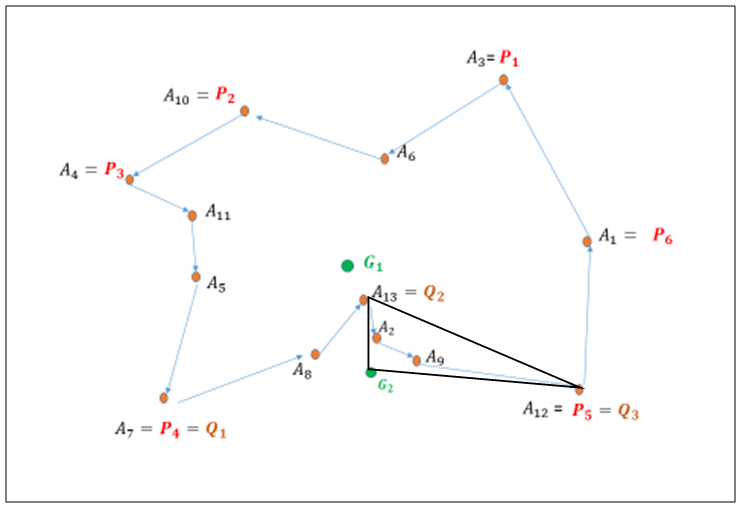Figure 8. Two points in the triangle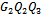-Thus the optimal path is as shown in Figure 9: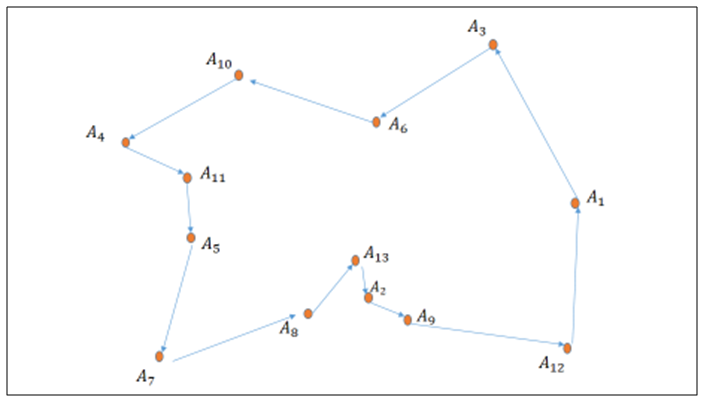Figure 9. Optimal path

### 4. Discussion

To understand the importance of the P versus NP problem let us imagine a world where P=NP. Technically we could have P = NP, but not have practical algorithms for most NP-complete problems. But suppose in fact we do have very quick algorithms for all these problems.
Many focus on the negative, that if P = NP then public-key cryptography becomes impossible. True, but what we will gain from P = NP will make the whole Internet look like a footnote in history.
Since all the NP-complete optimization problems become easy, everything will be much more efficient. Transportation of all forms will be scheduled optimally to move people and goods around quicker and cheaper. Manufacturers can improve their production to increase speed and create less waste.
Learning becomes easy by using the principle of Occam's razor—we simply find the smallest program consistent with the data. Near perfect vision recognition, language comprehension and translation and all other learning tasks become trivial. We will also have much better predictions of weather and earthquakes and other natural phenomenon.
P = NP would also have big implications in mathematics. One could find short, fully logical proofs for theorems but these proofs are usually extremely long. But we can use the Occam razor principle to recognize and verify mathematical proofs as typically written in journals. We can then find proofs of theorems that have reasonable length proofs say in under 100 pages. A person who proves P = NP would walk home from the Clay Institute not with \$1 million check but with seven (actually six since the Poincaré Conjecture appears solved).

### 5. Conclusions

The Travelling Salesman Problem is solvable in polynomial time, and consequently the polynomial solvability of NP-complete problems is stated.
The consequences of this solution are considerable in many areas: cryptology, computer science, mathematics, engineering, economics… The implications of the solution may make the resolution of other problems Millennium trivial.

### References

  Fortnow, L. The status of the P versus NP problem. Communications of the ACM, 52 (9): 78-86, 2009.  Cook, S. The complexity of theorem-proving procedures. In Proceedings of the 3rd ACM Symposium on the Theory of Computing, ACM, NY, 1971, 151-158.  Karp, R. Reducibility among combinatorial problems. Complexity of Computer Computations. R. Miller and J. Thatcher, Eds. Plenum Press, 1972, 85-103.  Levin, L. Universal'nyie perebornyie zadachi (Universal search problems: in Russian). Problemy Peredachi Informatsii, 9(3): 265-266, 1973.  Lawler, E.L., J.K. Lenstra, A.H.G. Kinnooy Kan, and D.B. Shmoys, eds, The Traveling Salesman Problem: A Guided Tour of Combinatorial Optimization, Wiley, 1985.  Dantzig, G.B., D.R. Fulkerson, and S.M. Johnson, Solution of a large-scale traveling- salesman problem, Operations Research, Vol. 2, 1954, pp. 393-4 10.  Nemhauser, G.L. and L.A. Wolsey, Integer and Combinatorial Optimization, Wiley, 1988.  Garey, M.R. and D.S. Johnson, Computers and Intractability: A Guide to the Theory of NP- Completeness, Freeman, San Francisco, 1979.  http://fr.openclassrooms.com/informatique/cours/theorie-des-collisions/formes-plus-complexes, 30/09/2014.